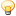# Discussione: IP / CIDR calculation in MySQL, PHP and JavaScript

1. ##IP / CIDR calculation in MySQL, PHP and JavaScript

Source: http://matthias.leisi.net/archives/1...avaScript.html

When writing the tools and website for the DNS Whitelisting project, http://www.dnswl.org/, I had to deal with a lot of IP calculation: data is stored in CIDR format, eg 127.0.0.1/32 to indicate a single IP address, 213.144.132.248/29 for a range of 213.144.132.248 to 213.144.132.254 and so on. RFC 1878 explains this notation in some detail.

Storing the dotted quad notation of an IP address would be pretty inefficient  the string is 15 characters long (four times up to three digits plus three dots), while it is technically just a nice display of a 32bit number. Additionally, comparing IP addresses (eg is this address within a given range) is a lot easier when done on the numeric representation since we can just use simple numeric comparison. In any case we need a way to convert between these two representations.

The CIDR notation itself is somewhat difficult to deal with  most humans will not be easily able to always know that the range 213.144.132.248/29 has 213.144.132.254 as its last IP address. We therefore also need a way to transform between CIDR and range notation. Unfortunately we need to do this in multiple environments, eg directly within the database (for certain batch jobs), in PHP (for regular backend operations) and in JavaScript (to avoid a server roundtrip on each transformation for the human reader).

MySQL

Luckily, MySQL has two functions to convert from dotted quad notation (127.0.0.1) to the corresponding decimal (2130706433), namely inet_aton and inet_ntoa (in case you wonder, thats ascii to numeric and vice versa). The Miscellaneous Functions chapter of the MySQL reference has the details.

select inet_aton('127.0.0.1'); and select inet_ntoa(2130706433); do all the byte twiddling for us. Note that this also works for insert and update statements, eg insert into ... inet_aton('127.0.0.1') .... The network mask (the "32" in "127.0.0.1/32") can be stored as a regular integer. Now that we know how to transform (2130706433, 32) into (127.0.0.1, 32), how do we transform a range (213.144.132.248, 29) into human readable 213.144.132.248 to 213.144.132.254? There we need to do some calculations of our own. If we have a network mask of /32, this means just one single host. A mask of /24 indicates 255 addresses, a /16 gives 65535 and a /8 gives 16777215 addresses. Yes, that has something to do with a number series involving a power of 2 and a minus 1, or to be more exact:
Codice:
`last IP = first IP + (2^(32-mask)) - 1`
In MySQL, this would translate into the following for (213.144.132.248, 29):
Codice:
`select inet_ntoa(inet_aton('213.144.132.248') + (pow(2,  (32-29))-1));`

PHP

We can apply the same logic to PHP as well. In PHP the functions to convert between dotted quad and numeric are called long2ip and ip2long (see the PHP Reference). The translation for (213.144.132.248, 29) would thus be:
Codice:
`\$lastip = \$firstip + pow(2, (32-\$mask)) - 1;`
Note the peculiarity of the long numerical type in PHP and how to get around that  the german de.comp.lang.php.* FAQ shows a workaround: http://www.php-faq.de/q/q-code-ip-bereich.html

JavaScript / ECMAScript

Unfortunately, JavaScript does not contain something similar to inet_aton or ip2long, so we have to build our own:
Codice:
```function ip2long(ip) {
var ips = ip.split('.');
var iplong = 0;
with (Math) {
iplong = ips<sup><a href="#fn0">0</a></sup>*pow(256,3)+ips<sup><a href="#fn1">1</a></sup>*pow(256,2)+ips<sup><a href="#fn2">2</a></sup>*pow(256,1)+ips<sup><a href="#fn3">3</a></sup>*pow(256,0)
}
return iplong;
}
function long2ip(l) {
with (Math) {
var ip1 = floor(l/pow(256,3));
var ip2 = floor((l%pow(256,3))/pow(256,2));
var ip3 = floor(((l%pow(256,3))%pow(256,2))/pow(256,1));
var ip4 = floor((((l%pow(256,3))%pow(256,2))%pow(256,1))/pow(256,0));
}
return ip1 + '.' + ip2 + '.' + ip3 + '.' + ip4;
}```
And the same power of two-thing to calculate the last IP:
Codice:
```function lastIP(ip, mask) {
return ip + (Math.pow(2, (32 - mask))-1);
}```

Bonus points

Dealing with user input is maybe the single most important security issue in web applications. We must therefore take care that we only pass proper IP addresses to any backend function. The easiest way to do this is through a regular expression  after all, we know exactly how a dotted quad representation of an IP address should look like (four times up to three digits, separated by dots). Thus the (Perl style) regex should look like
/^\d{1,3}\.\d{1,3}\.\d{1,3}\.\d{1,3}\$/
Validation of the numeric representation is of course trivial. In PHP:
Codice:
`if (!(is_numeric(\$ip)) { <b>kaboom</b>; }`
It is a good idea to always trim() user input before validation or processing (if leading/trailing whitespace is not significant).Rispondi Citando

#### Tag per Questa Discussione

cidr, javascript, mysql, php####Permessi di Scrittura

• Tu non puoi inviare nuove discussioni
• Tu non puoi inviare risposte
• Tu non puoi inviare allegati
• Tu non puoi modificare i tuoi messaggi
•